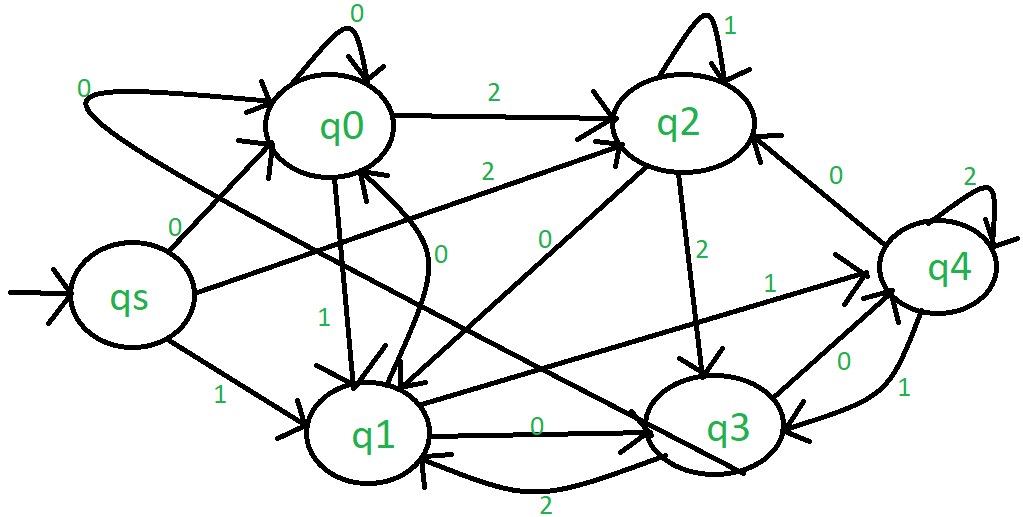GFG App
Open AppBrowser
Continue

# Finite State Machine to Determine the Ternary Number is Divisible 5

A finite state machine is a unquestionable model used to design and describe systems that have a finite add up of states, which change based on inputs. It is also noted as a finite automaton, and it is used in various fields such as computing device, science, mathematics, and engineering. The goal of this article is to define a finite put forward machine to determine whether a troika number (base 3) is severable by 5.

### Problem Statement

Given a ternary number, we need to design a finite state machine that will determine if the given number is divisible by 5 or not. A ternary come is a number system that has three digits, i.e., 0, 1, and 2, synonymous to a binary number system with two digits (0 and 1) or a decimal system with ten digits (0-9). We need to determine whether the precondition ternary add up is divisible by 5 or not using a finite state machine.

### Approach

To design a finite state machine, we need to follow careful steps, as mentioned below:

• Define the input alphabet: In this case, our input alphabet consists of three digits, i.e., 0, 1, and 2.
• Define the output alphabet: In this case, the output alphabet consists of deuce states, i.e., “Divisible by 5” and “Not divisible by 5.”

Now,

M=(Q , ∑ , η , δ , q0 , F)

Q = A set of non-empty finite states = {qs , q0 , q1 , q2 , q3 , q4}

= A set of non-empty finite input symbols = {0,1,2}

q0 = Initial state = {qs}

F = A non-empty finite set of final state = {q0}

δ = Q x ∑ ⇢ Q = {δ}

#### Transition Diagram:Transition diagram

#### Implementation:

We can implement the finite posit machine for determining the divisibility of a ternary number by 5 victimizations in any programming language or hardware description language (HDL) like Verilog or VHDL. Let’s take an example of implementing this machine in Verilog.

Verilog code for the finite state machine:

```module fsm_divisible_by_5(input clk, input rst,
input [1:0] input_digit, output reg output_state);
reg [2:0] current_state, next_state;

// Define the states
parameter S0 = 3'b000,
S1 = 3'b001,
S2 = 3'b010,
S3 = 3'b011,
S4 = 3'b100;

// Define the transition function
always@(posedge clk or posedge rst)
begin
if(rst)
current_state <= S0;
else
current_state <= next_state;
end

always@(input_digit)
begin
case(current_state)
S0: next_state = input_digit[1:0];
S1: next_state = {2'b10, 2'b01, 2'b00}[input_digit];
S2: next_state = {2'b01, 2'b10, 2'b11}[input_digit];
S3: next_state = {2'b10, 2'b01, 2'b00}[input_digit];
S4: next_state = {2'b01, 2'b10, 2'b11}[input_digit];
default: next_state = S0;
endcase
end

// Define the output state
always@(current_state)
begin
if(current_state == S0 || current_state == S5)
output_state = 1'b1; // Divisible by 5
else
output_state = 1'b0; // Not divisible by 5
end
endmodule```

The above Verilog code defines a module that takes an input time signal, a readjust signal, and a 2-bit input digit, and gives an yield put forward as the output. The input finger’s breadth represents the ternary digit (0, 1, or 2) of the given ternary number. The output submit wish be 1 if the given ternary number is separable by 5, otherwise 0.

#### Testing

To test the implemented finite state machine, we can provide different ternary numbers pool as input and control whether the output state is correct or not. For example, let’s take the ternion number 201. The output submit should be 1 as 201 is divisible by 5.

#### Verilog Testbench Code

```module fsm_divisible_by_5_tb;
reg clk, rst;
reg [1:0] input_digit;
wire output_state;

fsm_divisible_by_5 fsm(.clk(clk), .rst(rst),
.input_digit(input_digit),
.output_state(output_state));

initial begin
clk = 0;
forever #5 clk = ~clk;
end

initial begin
rst = 1;
input_digit = 2'b00;
#10 rst = 0;
#10 input_digit = 2'b10;
#10 input_digit = 2'b01;
#10 input_digit = 2'b01;
#10 \$display("Output State = %b", output_state);
#10 \$finish;
end
endmodule```

The above Verilog testbench code initializes the inputs and provides input digits to the finite state machine. It also simulates the clock signal and resets the machine at the beginning. After providing the input digits, it displays the output state and finishes the simulation.

When we run this testbench, the output should be:

`Output State = 1`

This output confirms that the given ternary number 201 is indeed divisible by 5.

### Conclusion:

In this article, we have discussed the problem of determining whether a given ternary number is divisible by 5 or not. We have explained the approach of using a finite state machine to solve this problem. We have also shown how to implement this finite state machine using Verilog and how to test it using a testbench. By following this approach, we can easily determine whether any given ternary number is divisible by 5 or not.

My Personal Notes arrow_drop_up